## Introduction

The generation and the manipulation of on-demand entangled photons are crucial in the emerging fields of quantum cryptography1 and quantum computation2. Hence, single semiconductor quantum dots (QDs) are considered potential sources of polarization-entangled photons through the radiative decay of biexcitons; a QD is initially excited to the biexciton state, and then it decays by a cascaded emission of two photons3. When the two exciton intermediate states are degenerate, the polarizations of the two photons are maximally entangled. However, they are not typically degenerated via the fine-structure splitting (FSS) owing to the asymmetries in the QD confining potential4, and its FSS energy (ΔFSS) must be less than the radiative linewidth (Γ). It is also necessary that the cascade emission takes place with a high probability, which is expected to increase the visibility of the two-photon coincidence and the degree of entanglement5. Until now, the sources that use this scheme have been limited to In(Ga)As QDs6,7,8,9,10,11,12 (with Γ of a few μeV)13, but the elongated shape and the strain in these QD systems induce a large in-plane anisotropy and a sizable ΔFSS (10–100 μeV)14. Because the typical ΔFSS observed for a QD exciton is usually larger than Γ, techniques to decrease ΔFSS must be used13,15,16,17.

Here we take a different approach that satisfies these requirements by using the naturally bright4,18,19 island QDs, made of a narrow GaAs quantum well (QW) with a monolayer thickness fluctuation4,20; the FSS is suppressed by applying an electric field (E) in the forward direction onto a Schottky diode structure. In addition to the lack of strain, which affects ΔFSS, GaAs island QDs provide an oscillator strength that is one order of magnitude larger than that of self-assembled In(Ga)As QDs; hence, they present a higher emission probability17,19,21 with a large Γ (23 μeV)4. This result arises because GaAs island QDs have a greater lateral extent area of the exciton than In(Ga)As QDs. Theoretically, GaAs island QDs are also expected to exhibit a smaller ΔFSS than In(Ga)As QDs because of the small electron–hole exchange energy, which is one order of magnitude smaller than that of self-assembled InAs QDs22. We observed a clear reduction of ΔFSS and a rotation of the exciton polarization axis caused by the electric field. At a certain value of the electric field, where ΔFSS is suppressed to below our detection limit, we obtained a high circular polarization degree of the exciton photoluminescence (PL) under a circularly polarized excitation. The polarization-dependent photon correlation measurements showed that applying electric fields to GaAs QDs makes it possible to manipulate the two-photon polarization state from the polarization-correlated state to the polarization-entangled state.

## Results

### GaAs island QDs in a Schottky device

GaAs QDs were grown on an n-GaAs (100) substrate by molecular beam epitaxy. The QDs were created in the regions of one monolayer thicker than the other regions, which were formed during a 2-min growth interruption on both sides of the GaAs QW. Figure 1a,b shows the fabricated Schottky diode structure and the simulation of the band diagram, respectively. No cavity structure was used to enhance the light-collection efficiency. An independent time-resolved μ-PL measurement was performed where the excitonic lifetime was found to be less than 300 ps, which reflects the fast radiative emission from our GaAs dots. We applied a forward bias voltage between the top gate metal contact and the bottom n-GaAs substrate (see Methods). The forward bias current (I) is quite small (I<5 nA) owing to the presence of a thick Al0.3Ga0.7As barrier, which inhibits large currents from flowing through the device (Fig. 1c). Low temperature (T=4 K) PL spectra are shown in Figure 1d as a function of E. The excitation and collection of PL was conducted through a 200-nm metal aperture, which was opened by electron beam lithography. For all measurements, we selected the excitation wavelength of 735–740 nm, which is about 40–50 meV higher than the exciton emission energy (approximately one longitudinal optical phonon energy of GaAs). Such an excitation wavelength is suitable to efficiently supply the photo-generated carriers to the QDs via phonon scattering.Figure 1: Fabricated Schottky diode structure and QD photoluminescence characteristics on applying an electric field.

### Photoluminescence under applied electric fields

The exciton PL emissions from two representative dots (dot A and dot B as indicated in Fig. 1d) were observed with their redshift in the presence of the electric field. Here we focused on the result of dot A, where four emissions—an exciton (X), biexciton (XX), negatively charged exciton (X) and positively charged exciton (X+)—were detected. In addition to the linear and the superlinear responses of their PL intensities to the excitation power, the exciton and the biexciton emissions were assigned according to their opposite linear polarization (Fig. 1e). Thus, the exciton and the biexciton can be easily distinguished from X and X+. The PL intensity dropped by 45% when E increased from 0 to 29.2 kV cm−1, most probably owing to an increase of the field-assisted carrier tunnelling. Above E=29.2 kV cm−1, the PL became significantly weak and broadened, which makes it difficult to determine ΔFSS at E>30 kV cm−1 (at these fields, I increases progressively above 10 nA (Fig. 1c)). The observed dependence of the PL spectra on the electric field for other dots was similar to that represented in Figure 1d.

### Changes in polarization and FSS with the electric field

The in-plane asymmetry of GaAs QDs is reflected in the polarization anisotropy exhibited by the integrated PL spectra of the exciton and the biexciton. By carefully following the evolution of the exciton and the biexciton PL energies with the polarization angle, we obtained a clear behaviour that could be fitted with a sinusoidal function, from which we extracted ΔFSS. The polarization axis of the PL was determined by the angle that represents the maximum splitting energy, and ΔFSS was measured with an accuracy of 2–3 μeV. Figure 2a shows a clear nonlinear change of ΔFSS with the electric field, where ΔFSS decreased from 38 μeV at E=0 to below our detection limit when the field approached 30 kV cm−1. We studied five quantum dots; three of them showed a similar FSS suppression behaviour with the increase in the electric field. Moreover, on tuning ΔFSS over the measured range of the electric field, we observed a clear rotation of the exciton polarization axis. Figure 2b summarizes the relationship between the electric field and ϕ, which describes the orientation of the exciton polarization axis relative to the crystal axis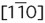. We found that as the field increased, the exciton polarization axis continuously rotated from ϕ=90°, where it aligned with the crystal axis  at E=−45 kV cm−1, until it approached 45°, and it became independent of the polarization axis at E=29.2 kV cm−1, where ΔFSS was minimized within our detection limit (Fig. 2c). Similar results have recently been reported for InAs/GaAs QDs using a large electric field of up to a few hundred kV cm−1 (ref. 12).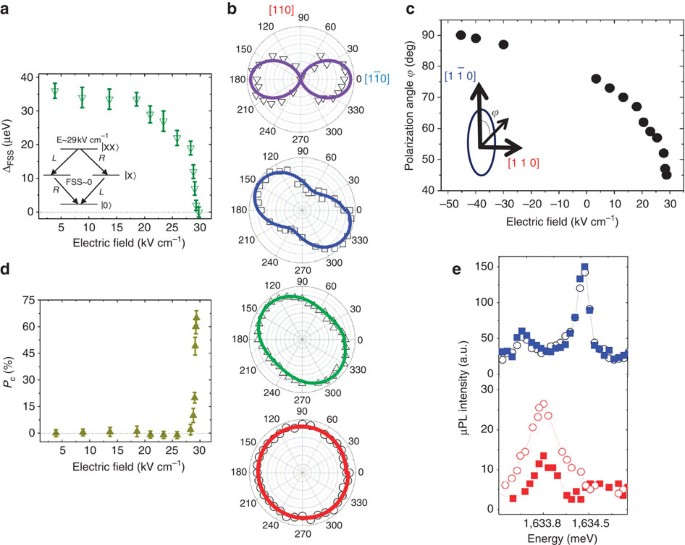Figure 2: Electric field-dependence of fine-structure splitting energy and QD exciton polarization.

To confirm that ΔFSS approaches zero with the increase in the electric field, the QD exciton was excited by circularly polarized light with an energy of 40 meV above the exciton PL transition energy, and the exciton spin polarization was monitored by its PL circular polarization. Such one-longitudinal optical-phonon-assisted circularly polarized excitation induces a coherent superposition of both fine structure components of the exciton, which are split by ΔFSS. Analogous to the well-known Hanle effect, the degree of the exciton PL circular polarization (Pc) is expected to increase when ΔFSS approaches zero23. Figure 2d shows the evolution of Pc with the electric field. The polarized spectra of the exciton were plotted in Figure 2e for two different electric field strengths (E=23 and 29.2 kV cm−1). When the field strength increased, a strong increase in Pc (65%) was observed, indicating that ΔFSS was greatly reduced at E=29.2 kV cm−1 compared with that at E=23 kV cm−1.

### Quantum-confined Stark spectroscopy

Now, we discuss the influence of the electric field on ΔFSS based on the QD exciton and biexciton Stark shift. A quantum confined Stark effect can introduce changes in the QD Coulomb interactions by modifying the overlap of the carriers' wavefunctions. Experimentally, we found that the emissions exhibited a quadratic shift according to the equation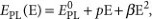where EPL is the PL energy, and p and β are the permanent dipole and the polarizability of the state, respectively24. The solid lines in Figure 3 show the results of the parabolic fit to the exciton and biexciton emission energies, from which we extracted p/e=−0.28±0.02 nm and β=−1.32±0.008 μeV/(kVcm−1)2 for the exciton. A negative value of p indicates a permanent dipole moment along the −z direction; the hole wavefunction is located below the electron wavefunction (Fig. 1a).

In Figure 3, we plotted the electric-field dependence of the biexciton binding energy BXX, which is defined as the energy difference between the exciton and the biexciton emissions. A systematic reduction in BXX was observed. In a simple model, BXX can be expressed as: BXX=(−2Jeh+Jee+Jhh), where Jeh is the electron–hole Coulomb interaction, and Jee and Jhh are the electron–electron and the hole–hole Coulomb interactions, respectively. At E=0, a small spatial separation between the electron and the hole wavefunctions exists, giving rise to a permanent dipole moment. Because positive E and p are in the same direction as depicted in Figure 1a, the wavefunction separation increased with an increasing electric field, which led to a reduction in BXX by decreasing Jeh. A decrease in BXX with the reduction of ΔFSS was previously reported for annealed InAs self-assembled QDs25. Although further study is required to understand the BXX reduction with the field, our results suggest that a positive electric field manipulates the electron–hole separation along the growth direction, and simultaneously modifies their lateral confinement; thus, the electric field enables the exciton dipole to rotate in the sample plane. Modulation of the exciton wavefunction by the electric field results in a decrease in the QD electron–hole overlap and a nonlinear change in the long-range component of the electron–hole exchange interaction, which strongly influences the FSS26. Although the electron–hole separation is expected at negative fields, we observed a sizable value of ΔFSS (=60 μeV) at E<−30 kV cm−1 (Fig. 2b), indicating the absence of a significant lateral modification of the exciton wavefunction at such field strengths. This observation is consistent with the lack of in-plane rotation of the exciton dipole within this electric field range (Fig. 2b,c). The polarity dependence may be related to the asymmetric nature of the two confining interfaces in our GaAs island QD27. It should be noted that the electric field dependence of ΔFSS in this case is qualitatively different from that of InAs/GaAs QDs, in which ΔFSS decreases linearly with the application of large reverse electric fields12. This difference might result from the 'weak'4,28 lateral confining potential of our GaAs island QDs compared with the 'strong' lateral confinement of InAs/GaAs QDs (as a result, the carriers' wavefunctions in GaAs island QDs will be more sensitive to the applied electric fields than those of InAs/GaAs QDs).

### Polarization-resolved photon correlation measurements

The suppression of the FSS by the electric field enabled us to examine the generation of polarization-entangled photons from the same dot. This generation relies on the biexciton–exciton cascaded emission, which can proceed along two paths as shown in the inset of Figure 2a: with a left-circularly-polarized (L) biexciton photon followed by a right-circularly-polarized (R) exciton photon, or vice versa. As ΔFSS approaches 0, it is impossible to distinguish which path the system has been followed, and the two photons should be emitted in the Bell state:Alternatively, the Bell state can be rewritten1,9 in the linear polarization basis as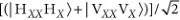or in the diagonal polarization basis as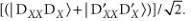The first clear signature of the polarization-entangled photons from our GaAs island dot was observed in polarization-resolved photon correlation measurements (Fig. 4a). A clear photon bunching occurred for the collinearly polarized exciton and the biexciton photons emitted in the same cascade at zero delay time in the linear (VV) and the diagonal bases (DD), whereas in the circular basis, the bunching was observed only for the counter-circularly polarized (RL) photons. This result is expected for entangled photon pairs in the Bell state because the two-photon wavefunctions can be expressed as a superposition of the collinearly, co-diagonally or cross-circularly-polarized photon pairs3. The degree of entanglement was quantified using the degree of correlation (see Methods) (Fig. 4b), where the entanglement fidelity f+ of the emitted photons projected onto the maximally entangled state was determined directly by combining the correlations measured in the linear, the diagonal and the circular polarization bases29. We found that the peak at the zero time delay yields a fidelity f+=0.72±0.05 (Fig. 4c) without any background light subtraction, which exceeds the threshold of 0.5 for a source emitting classically polarization-correlated states. Thus, the result proves that entangled photons were generated. Figure 4d depicts the results of the polarization-resolved photon correlation measurements when the electric field decreased to 18 kV cm−1, where the corresponding ΔFSS was 30 μeV. Under these conditions, there is a high probability of generating a pair of photons with the same linear polarization, as shown in Figure 4e. No significant polarization correlation was observed in the diagonal and the circular bases. Hence, the fidelity under these conditions is below 0.5 (Fig. 4f), which shows that the two-photon state at 18 kV cm−1 is classically polarization correlated.

## Discussion

The above results demonstrate that the two-photon state emitted from the same GaAs QD can be manipulated from the polarization-correlated state to a polarization-entangled state by applying a vertical electric field. Because these GaAs island QDs emit light at a short wavelength (~750 nm), they will be useful for efficient detection of photons with silicon detectors. The reduction in ΔFSS for these dots, caused by the forward bias voltage, may also allow the fabrication of an efficient visible light-emitting diode, which can emit entangled photons with a high emission probability that is suitable for qubit-based optical30 and spin31 quantum computation applications.

## Methods

### Quantum dots and device fabrication

The sample consists of a 14-monolayer GaAs QW sandwiched between 25-nm-thick Al0.3Ga0.7As barriers and covered with a 50-nm-thick GaAs cap layer. A 2-min growth interruption was performed on both sides of the GaAs QW. This QW structure was grown on top of a 100-nm-thick undoped GaAs layer deposited on a 300-nm-thick n-GaAs layer (n=1.5×1017 cm−3). All layers were epitaxially grown by molecular beam epitaxy on an n-GaAs (100) substrate, which was used as a backside electrode. A Ti/Au film with an aperture of 200 nm in diameter was deposited on the sample surface to form a Schottky contact. The electric field is defined as E=(VgV0)/d, where Vg is the applied gate voltage, V0 is the Schottky barrier height (=0.93 V) obtained from independent photocurrent measurements, and d is the distance between the sample surface and the bottom n-doped GaAs layer (d=205 nm).

### Optical spectroscopy measurements

We focused the laser and collected the luminescence using a microscope objective lens with a numerical aperture of 0.4. A continuous wave and pulsed (6 ps) Ti:Al2O3 laser was used for the excitation. The PL spectra were analysed by using a 55-cm-long monochromator coupled to an N2-cooled silicon charge-coupled device detector. A spectral notch filter was used to suppress the laser emission. Most of the QDs across the sample had line widths of ~120 μeV and were limited mainly by our set-up resolution. In the photon correlation measurements, the Hanbury Brown and Twiss (HBT) set-up was used to generate the photon correlation histograms with various polarization combinations. The collected PL was divided into two orthogonal detection arms using a 50/50 nonpolarizing pellicle beam splitter. Each arm of the HBT set-up was equipped with a single monochromator to filter the PL emission individually, in addition to a high-sensitivity avalanche photodiode. The electrical pulses from the two avalanche photodiodes were used to start and to stop a time-to-amplitude converter. The data within ±2.5 ns of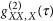were integrated to extract the total number of counts. For all optical measurements, the sample temperature was T=4 K. A combination set of half-wave and quarter-wave plates was inserted in the collection path of the HBT set-up for proper polarization state selection. No background subtraction or filtering was performed. The fidelity f+ to the Bell state was calculated by measuring the correlation function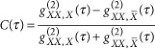for each polarization basis of the linear, diagonal and circular types29, whereand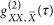are the second-order correlation functions when a biexciton photon is detected with a co-polarized and a cross-polarized exciton photon, respectively. The f+ is expressed as: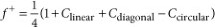where Clinear, Cdiagonal and Ccircular are the degrees of polarization correlation between the two emitted photons in the linear, the diagonal and the circular bases, respectively.